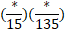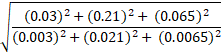# Quiz Discussion

The number of trees in each row of a garden is equal to the total number of rows in the garden. After 111 trees have been uprooted in a storm, there remain 10914 trees in the garden. The number of rows of trees in the garden is = ?

Course Name: Quantitative Aptitude

• 1] 100
• 2] 105
• 3] 115
• 4] 125
##### Solution
No Solution Present Yet

#### Top 5 Similar Quiz - Based On AI&ML

Quiz Recommendation System API Link - https://fresherbell-quiz-api.herokuapp.com/fresherbell_quiz_api

# Quiz
1
Discuss

How many perfect squares lie between 120 and 300 ?

• 1] 5
• 2] 6
• 3] 7
• 4] 8
##### Solution
2
Discuss

If $$\sqrt 5 = 2.236,$$   then the value of $$\frac{{\sqrt 5 }}{2} - \frac{{10}}{{\sqrt 5 }} + \sqrt {125}$$   is equal to :

• 1] 5.59
• 2] 7.826
• 3] 8.944
• 4] 10.062
##### Solution
3
Discuss

The square root of $$\left( {{{272}^2} - {{128}^2}} \right)$$  is = ?

• 1] 144
• 2] 200
• 3] 240
• 4] 256
##### Solution
4
Discuss

$$1728 \div \root 3 \of {262144} \times ? - 288$$      = 4491

• 1] 148
• 2] 156
• 3] 173
• 4] 177
##### Solution
5
Discuss

For what value of * the statement= 1 is true?

• 1] 15
• 2] 25
• 3] 35
• 4] 45
##### Solution
6
Discuss

If $$\sqrt {33} = 5.745{ \text{}}$$   then which of the following values is approximately $$\sqrt {\frac{3}{{11}}} { \text{ ?}}$$

• 1] 1
• 2] 6.32
• 3] 0.5223
• 4] 2.035
##### Solution
7
Discuss

Square root of 64009 is

• 1]

253

• 2]

347

• 3]

363

• 4]

803

##### Solution
8
Discuss

The value ofis ?

• 1]

0.1

• 2]

10

• 3]

$${10^2}$$

• 4]

$${10^3}$$

##### Solution
9
Discuss

The value of $$\sqrt {0.01} { \text{ + }} \sqrt {0.81} { \text{ + }} \sqrt {1.21} { \text{ + }} \sqrt {0.0009}$$  is = ?

• 1] 2.03
• 2] 2.1
• 3] 2.11
• 4] 2.13
##### Solution
10
Discuss

$$\left( {\frac{{\sqrt {625} }}{{11}} \times \frac{{14}}{{\sqrt {25} }} \times \frac{{11}}{{\sqrt {196} }}} \right){\kern 1pt}$$     is equal to :

• 1] 5
• 2] 6
• 3] 8
• 4] 11
# Quiz